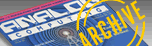`A.N.A.L.O.G. ISSUE 69 / FEBRUARY 1989 / PAGE 6`Is there something about the Mandelbrot set that turns the mind to mush?     Actually, James Greco's article (September '88) is in many ways an admirable effort. He handles real and imaginary numbers and squaring complex numbers very well. His explanation of where the different colors come from in plotting the environs of the Mandelbrot set is really fine.     The problem arises from an incorrect formula for the function that generates the Mandelbrot set. The formula, given twice on page 20, should be Z < - Z ^ 2+U. (The square was on the wrong Z in the article.) This notation is analogous to the BASIC line LET Z =Z ^ 2+U. It means "replace Z by its current value squared plus U."     The only trouble is that Z and U are supposed to be complex numbers and BASIC doesn't do complex-number arithmetic on its own. However, if you follow Greco's explanation (page 19), you will see how to square a complex number using high-school algebra. Here is the general formula: (a+bi) ^2=a ^2-b ^ 2+2abi, where i is the famous square root of -1. In the program, the squaring and adding operations are carried out in Line 20. And in spite of the error in the article, the program does the calculation exactly right.     But here is the key point that is missed because of the notation mistake: What makes the Mandelbrot set so complex is that it is not a pure squaring operation. If you square a number, take the result and square it again, and so on, and plot the results the way it is done in plotting the Mandelbrot set, you get a far simpler looking graph. (I think it would be a series of concentric colored rings enclosing a black disk, themselves surrounded by a monochrome background.) The immense complexity comes from combining squaring and adding. -Jerry Bridgman Madison, WI